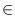Next: Real Circles ( real_circle Up: Basic Data Types for Previous: Real Rays ( real_ray   Contents   Index

# Straight Real Lines ( real_line )

Definition

An instance l of the data type real_line is a directed straight line in the two-dimensional plane.

#include < LEDA/geo/real_line.h >

Types

 real_line::coord_type the coordinate type (real). real_line::point_type the point type (real_point).

Creation

 real_line l(const real_point& p, const real_point& q) introduces a variable l of type real_line. l is initialized to the line passing through points p and q directed form p to q. real_line l(const real_segment& s) introduces a variable l of type real_line. l is initialized to the line supporting segment s. real_line l(const real_ray& r) introduces a variable l of type real_line. l is initialized to the line supporting ray r. real_line l(const real_point& p, const real_vector& v) introduces a variable l of type real_line. l is initialized to the line passing through points p and p + v. real_line l introduces a variable l of type real_line. l is initialized to the line passing through the origin with direction 0. real_line l(const line& l1, int prec = 0) introduces a variable l of type real_line initialized to the line l1. (The second argument is for compatibility with rat_line.) real_line l(const rat_line& l1) introduces a variable l of type real_line initialized to the line l1.

Operations

 real_point l.point1() returns a point on l. real_point l.point2() returns a second point on l. real_segment l.seg() returns a segment on l. bool l.is_vertical() returns true iff l is vertical. bool l.is_horizontal() returns true iff l is horizontal. real l.sqr_dist(const real_point& q) returns the square of the distance between l and q. real l.distance(const real_point& q) returns the distance between l and q. int l.orientation(const real_point& p) returns orientation(l.point1(), l.point2(), p). real l.slope() returns the slope of l. Precondition l is not vertical. real l.y_proj(real x) returns p.ycoord(), where pl with p.xcoord() = x. Precondition l is not vertical. real l.x_proj(real y) returns p.xcoord(), where pl with p.ycoord() = y. Precondition l is not horizontal. real l.y_abs() returns the y-abscissa of l (l.y_proj(0)). Precondition l is not vertical. bool l.intersection(const real_line& g, real_point& p) if l and g intersect in a single point this point is assigned to p and the result is true, otherwise the result is false. bool l.intersection(const real_segment& s, real_point& inter) if l and s intersect in a single point this point is assigned to p and the result is true, otherwise the result is false. bool l.intersection(const real_segment& s) returns true, if l and s intersect, false otherwise. real_line l.translate(real dx, real dy) returns l translated by vector (dx, dy). real_line l.translate(const real_vector& v) returns l translated by vector v. Precondition v.dim() = 2. real_line l + const real_vector& v returns l translated by vector v. real_line l - const real_vector& v returns l translated by vector - v. real_line l.rotate90(const real_point& q, int i=1) returns l rotated about q by an angle of i x 90 degrees. If i > 0 the rotation is counter-clockwise otherwise it is clockwise. real_line l.reflect(const real_point& p, const real_point& q) returns l reflected across the straight line passing through p and q. real_line l.reverse() returns l reversed. real_segment l.perpendicular(const real_point& p) returns the segment perpendicular to l with source p. and target on l. real_point l.dual() returns the point dual to l. Precondition l is not vertical. int l.side_of(const real_point& p) computes orientation(a, b, p), where ab and a and b appear in this order on line l. bool l.contains(const real_point& p) returns true if p lies on l. bool l.clip(real_point p, real_point q, real_segment& s) clips l at the rectangle R defined by p and q. Returns true if the intersection of R and l is non-empty and returns false otherwise. If the intersection is non-empty the intersection is assigned to s; it is guaranteed that the source node of s is no larger than its target node.

Non-Member Functions

 int orientation(const real_line& l, const real_point& p) computes orientation(a, b, p) (see the manual page of real_point), where ab and a and b appear in this order on line l. int cmp_slopes(const real_line& l1, const real_line& l2) returns compare(slope(l1), slope(l2)).Next: Real Circles ( real_circle Up: Basic Data Types for Previous: Real Rays ( real_ray   Contents   Index